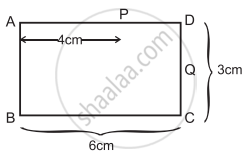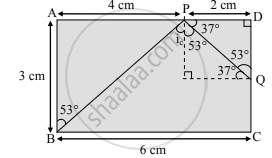Advertisement Remove all ads

# Solve Numerical example. Figure below shows the section ABCD of a transparent slab. There is a tiny green LED light source at the bottom left corner B. - Physics

Numerical

Solve Numerical example.

Figure below shows the section ABCD of a transparent slab. There is a tiny green LED light source at the bottom left corner B. A certain ray of light from B suffers total internal reflection at nearest point P on the surface AD and strikes the surface CD at point Q. Determine refractive index of the material of the slab and distance DQ. At Q, the ray PQ will suffer partial or total internal reflection? [Angles of the most popular Pythagorean triangle of sides in the ratio 3:4:5 are nearly 37°, 53°, and 90°]Advertisement Remove all ads

#### SolutionAs the light ray undergoes total internal reflection at P, the ray BP may be incident at a critical angle. For a Pythagorean triangle with sides in ratio 3: 4: 5 the angle opposite to side 3 units is 37° and that opposite to 4 units is 53°.

Thus, from the figure, we can say, in ΔBAP,
∠ABP = 53°
∴ ∠BPN - ic = 53°
∴ nglass = 1/(sin"i"_"c")=1/sin(53°)≈1/0.8=5/4

∴ Refractive index (n) of the slab is 5/4.

From symmetry, ΔPDQ is also a Pythagorean triangle with sides in ratio QD: PD: PQ = 3: 4: 5.
∴ PD = 2 cm ⇒ QD = 1.5 cm.
As the critical angle is ic = 53° and the angle of incidence at Q, ∠PQN = 37° is less than the critical angle, there will be a partial internal reflection at Q.

The refractive index of a material is 5/4. The ray PQ will suffer partial internal reflection at Q.

Concept: Reflection
Is there an error in this question or solution?
Advertisement Remove all ads

#### APPEARS IN

Balbharati Physics 11th Standard Maharashtra State Board
Chapter 9 Optics
Exercises | Q 4. (vii) | Page 187
Advertisement Remove all ads
Advertisement Remove all ads
Share
Notifications

View all notifications

Forgot password?